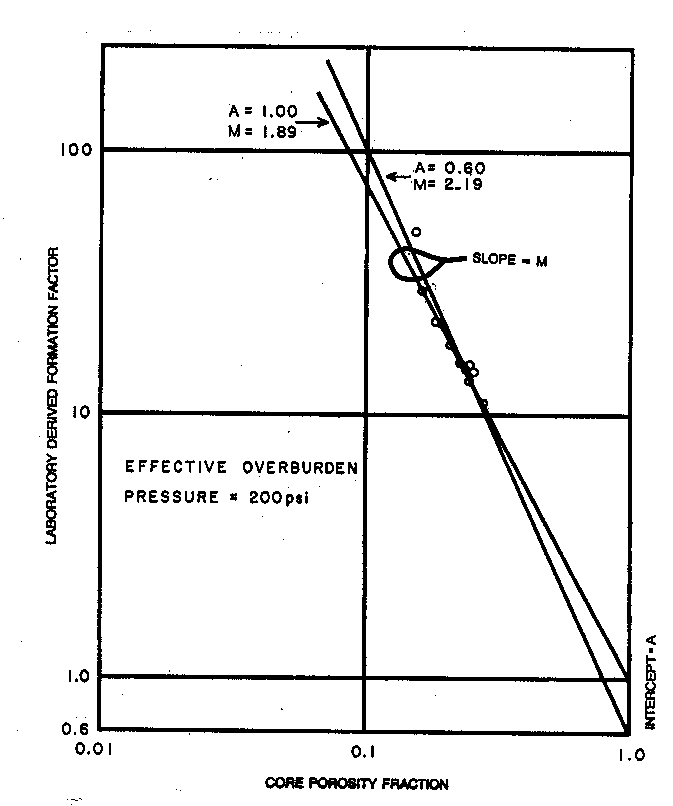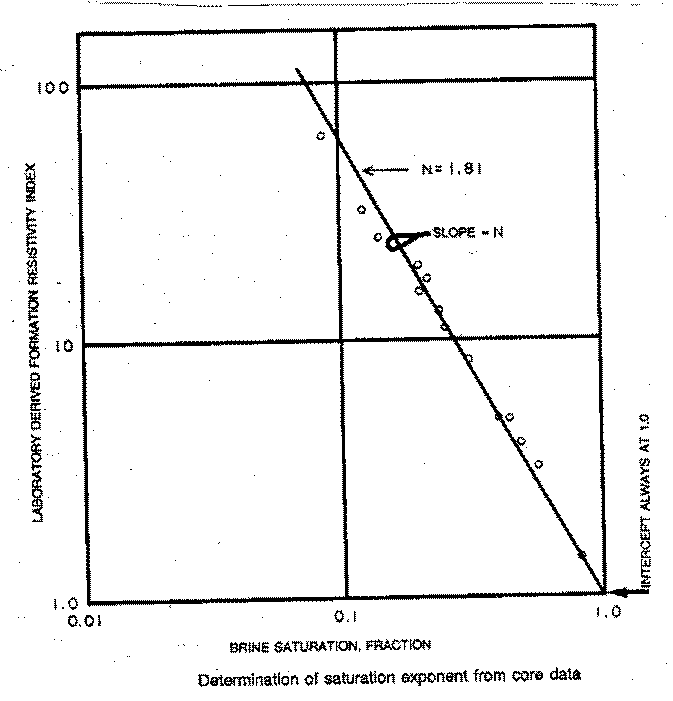ARCHIE'S First LAW -- Cementation Exponent "M"
Most saturation methods rely on work originally done by Gus Archie in 1940-41. Archie's Laws have since been extended to include shaly sands and carbonates with electrical properties that vary with pore geometry. See
Resistivity in Rocks and Electrical Properties A, M, N for details

He found from laboratory studies that, in a shale free, water filled rock, the Formation Factor (F) was a constant defined by:
1: F = R0 / Rw

He also found that F varied with porosity:
2: F = A / (PHIt ^ M)

For a tank of water, R0 = Rw. Therefore F = 1. Since PHIt = 1, then A must also be 1.0 and M can have any value. If porosity is zero, F is infinite and both A and M can have any value. However, for real rocks, both A and M vary with grain size, sorting, and rock texture. The normal range for A is 0.5 to 1.5 and for M is 1.7 to about 3.2. Archie used A = 1 and M = 2. In fine vuggy rock, M can be as high as 7.0 with a correspondingly low value for A. In fractures, M can be as low as 1.1. Note that R0 is also spelled Ro in the literature. This relationship is illustrated below:Formation Factor versus Porosity plot to find saturation parameters A and M.

Archie initially proposed that A = 1.0, M = 2.0, and N = 2.0; later it was found that these values varied with rock type. When electrical properties are available, they should be used, provided they fall within reasonable ranges and the data set is large enough to be valid. Data is usually presented in tabular as well as graphical form, as shown below.

The slope of the best fit line through the formation factor data is the cementation exponent, M. The best fit line can be forced through the origin (a pinned line) which makes the tortuosity factor A = 1.0 exactly. The intercept of the best fit (un-pinned) line will give A; in this example A = 0.60. Data should be grouped by rock type, porosity type, or mineralogy, before the best fit lines are determined.ARCHIE'S SECOND LAW -- saturation Exponent "n"
For rocks with both hydrocarbon and water in the pores, he also defined the term Formation Resistivity Index ( I ) as:
3: I = Rt / R0
4: Sw = ( 1 /
I ) ^ (1 / N)

Archie used an N of 2 and the usual range is from 1.3 to 2.6, depending on rock texture. It is often taken to equal M, but this is not supported by core data in all cases. Rearrangement of these four equations give the more usual Archie water saturation equation  This relationship is illustrated below:Resistivity Index versus Water Saturation to determine saturation exponent N

The value for the saturation exponent N is usually found in the laboratory as shown above. It is a plot of resistivity index ( I ) versus water saturation. Several partial saturations are taken on each core plug and N is determined from the slope of the line through these points. N can be varied by defining lithofacies for each core plug and relating this to some log signature.

The best fit line on this plot is always pinned at the origin, since resistivity index must equal 1.0 when SW = 1.0, by definition.

Shale corrections are applied by adding a shale conductivity term with an associated shale porosity and shale formation factor relationship. Numerous authors have explored this approach, leading to numerous potential solutions for water saturation.

Where:
A = tortuosity exponent (unitless)
M = cementation exponent (unitless)
N = saturation exponent (unitless)
F = formation factor (unitless)

I  = resistivity index (unitless)
PHIt = total  porosity (fractional)
R0 = resistivity of a water filled rock (ohm-m)
Rt = resistivity of a rock at irreducible water saturation (ohm-m)
Rw = water resistivity at formation temperature (ohm-m)

RECOMMENDED PARAMETERS:
for carbonates A = 1.00  M = 2.00  N = 2.00  (Archie Equation as first published)
for sandstone  A = 0.62  M = 2.15  N = 2.00  (Humble Equation)
A = 0.81  M = 2.00  N = 2.00  (Tixier Equation - simplified version of Humble Equation)
NOTE: N is often lower than 2.0

For quick analysis use carbonate values. Values for local situations should be developed from special core data. Results will always be better if good local data is used instead of traditional values, such as those given above.

Asquith (1980 page 67) quoted other authors, giving values for A and M, with N = 2.0, showing the wide range of possible values:

Average sands              A = 1.45  M = 1.54
Shaly sands                  A = 1.65  M = 1.33
Calcareous sands         A = 1.45  M = 1.70
Carbonates                   A = 0.85  M = 2.14
Pliocene sands S.Cal.  A = 2.45  M = 1.08
Miocene LA/TX             A = 1.97  M = 1.29
Clean granular             A = 1.00  M = 2.05 - PHIe

Water saturation can be calibrated by comparing log analysis results with water saturation from capillary pressure data on core samples, and in some cases from oil base cores.

Page Views ---- Since 01 Jan 2015
Copyright 2023 by Accessible Petrophysics Ltd.
CPH Logo, "CPH", "CPH Gold Member", "CPH Platinum Member", "Crain's Rules", "Meta/Log", "Computer-Ready-Math", "Petro/Fusion Scripts" are Trademarks of the Author